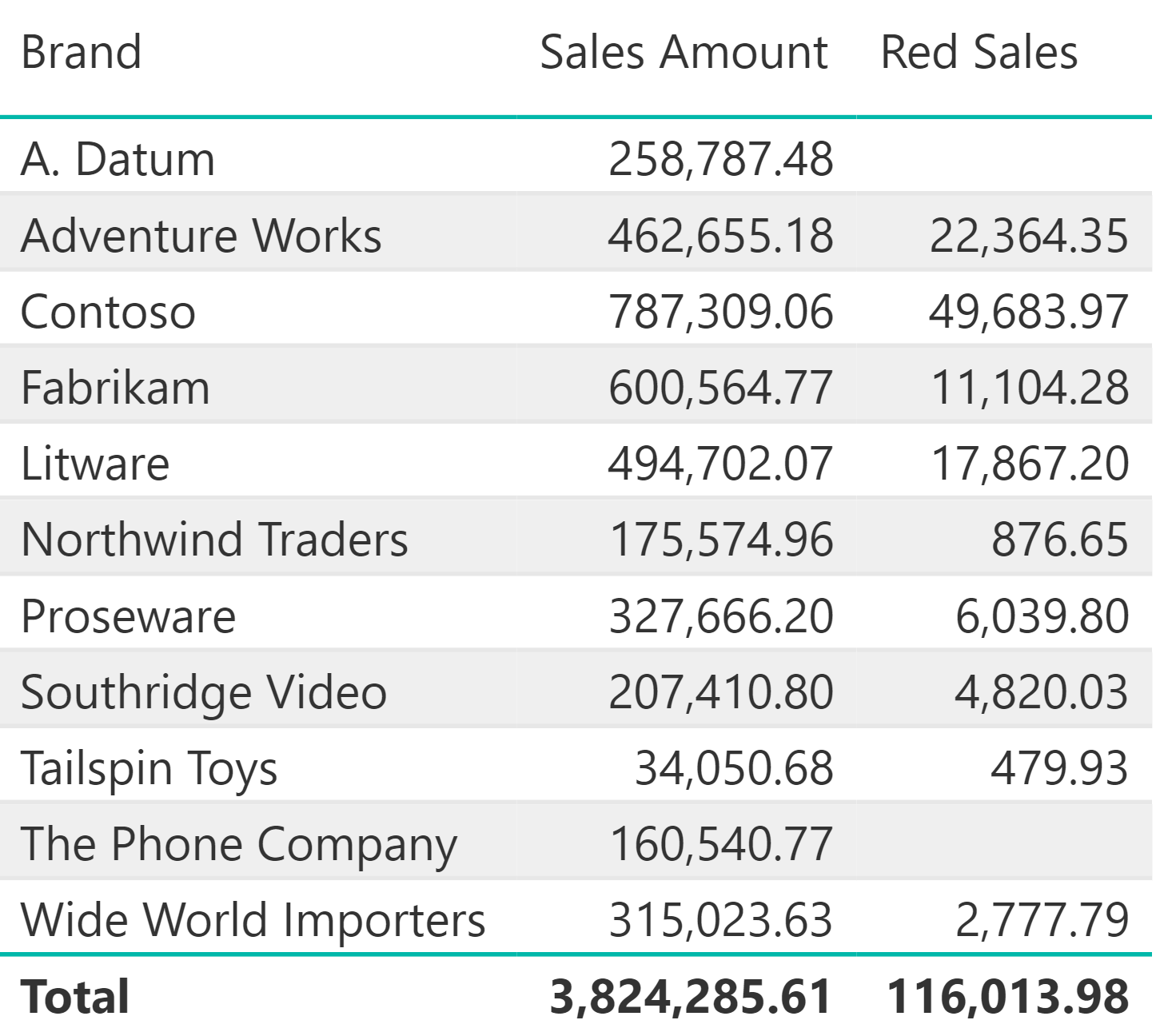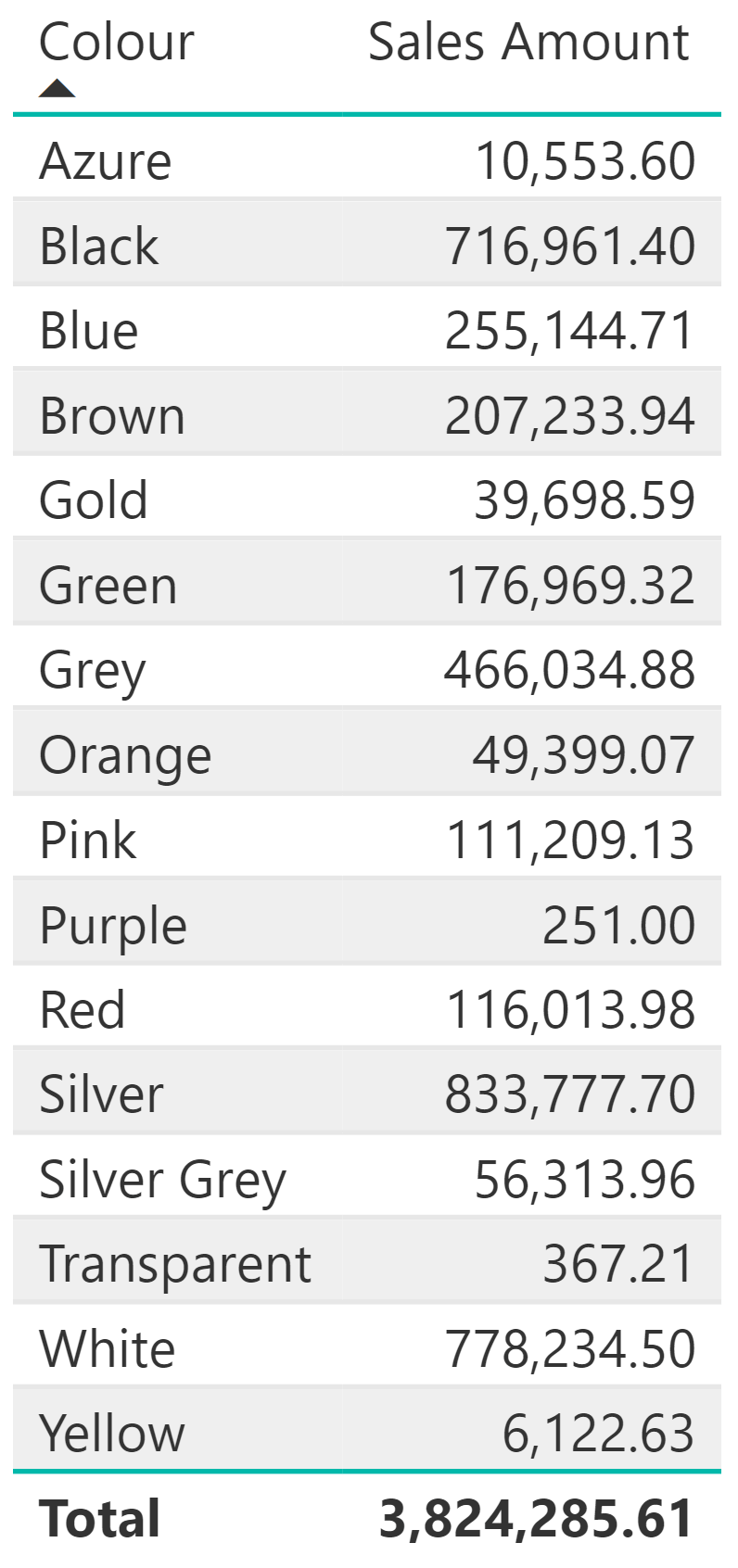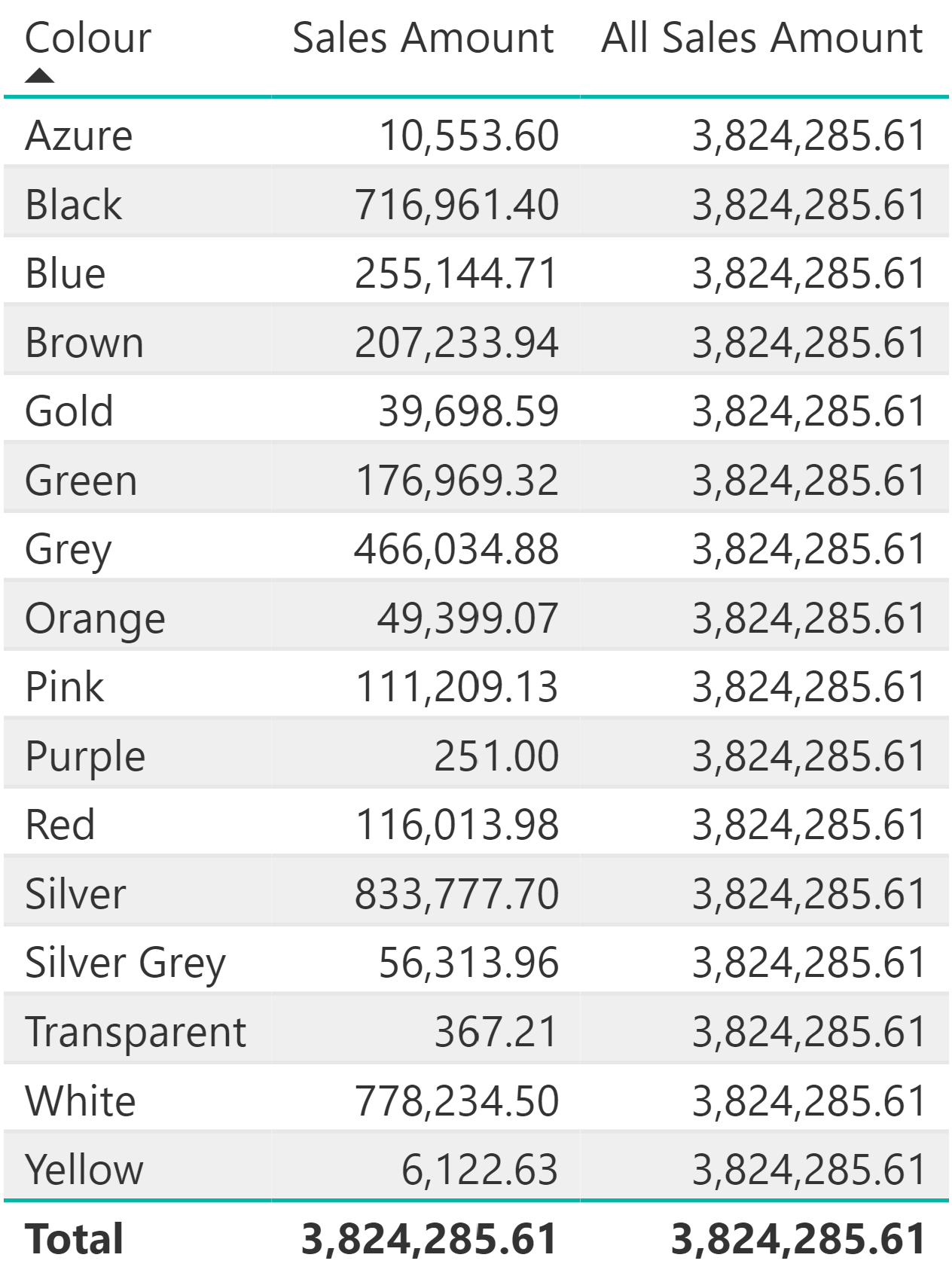## What are table functions?

Table functions are functions in DAX that return a table. These are virtual tables inside of Power BI that are created using DAX functions, rather than tables that are physically within the data model. This blog post will explore two of the most common table functions – `FILTER` and `ALL`.

## FILTER

The `FILTER` table function is useful if you want to filter a table. The `FILTER` function returns a sub-set of a table. To use the `FILTER` function, you first specify a table name, followed by a condition. The condition is evaluated row by row on top the specified table and only the rows satisfying the condition will be returned as a result. So, the `FILTER` function acts as both a table function and an iterator.

In order to illustrate how the `FILTER` function works, we will create a new calculated table that is filtered to only return products from the 'Product' table with a colour of red.

``````Red Products = FILTER ('Product', 'Product'[Colour] = “Red”)
``````

Here, the `FILTER` function will evaluate every single row of the 'Product' table. For each row, if the 'Colour' column has a value of 'Red', this row will be included in the new calculated table as shown below.Whilst this example illustrates how the `FITLER` function operates, in practice, you typically want to use a table function to create a temporary table that is scanned and iterated by some other function. In other words, `FILTER` is useful when you want to return the result of a calculation which is done over a subset of values from a table. For example, we can create a measure that uses `FILTER` to show only the sales of products which are red.

``````Red Sales =
SUMX (
FILTER (
Sales,
RELATED ( 'Product'[Colour] ) = "Red"
),
Sales[Quantity] * Sales[Net Price]
)
``````

Here, `FILTER` is working on the 'Sales' table, but the 'Sales' table does not contain the product colour, so we want to use the function `RELATED`. `RELATED` gives you access to columns from other tables that have a relationship with the current table you are scanning.

For each sale in the 'Sales' table, the `RELATED` function looks up the 'Colour' value in the 'Product' table. The result of this lookup is used by the `FILTER` function to identify and return rows where the 'Colour' column has a value of 'Red'. This temporary table is then scanned and iterated by `SUMX` to calculate the `Sales[Quantity] * Sales[Price]` for each row in the table. This computation calculates the sales amount for only red products.

This new `Red Sales` measure has been added to the table below to create a new column which shows the red sales for different brands.## ALL

Now, we shall explore the next table function in this blog post - `ALL`. `ALL` returns all the rows in a table, or all the values in a column, ignoring any filters that may have been applied.

In the table below, we have the colour of each product and we have the sales amount which has been calculated via the following measure:

``````Sales Amount = Sales[Quantity] * Sales[Net Price]
```````ALL` is useful if you want to compute percentages and ratios. If I want to see the percentage of red sales against all of the product sales I will need to compute the denominator of this ratio – the grand total of all the sales. We can do this using a new measure:

``````All Sales Amount = SUMX ( ALL ( Sales ), Sales[Quantity] * Sales[Net Price])
``````

Here, `SUMX` requires the result of `ALL` Sales. `ALL` ignores any filter that is applied in the report and returns all of the sales. `SUMX` iterates over the whole table computing Quantity multiplied by Net Price, even if there are filters in place (such as the filter of 'Colour' in the example below). Whenever you use `ALL`, any filter gets removed and you always compute the same number for all of the cells, as we can see in the table below.Now we have the denominator `All Sales Amount`, we can remove this measure from our table and create our percentage sales amount calculation:

``````Sales % = [Sales Amount] / [All Sales Amount]
``````

The `Sales %` measure can be added into the table as a new column which displays the percentage of sales of each colour over the grand total of sales.## Conclusion

In this blog post we have seen how the `FILTER` table function can be used to filter a table and is useful when you want to return the result of a calculation over a subset of values. We have also seen how the `ALL` table function can be used to return all rows of a table or values of a column, ignoring any filters applied and is useful when computing percentages and ratios.

### Jessica Hill

#### Apprentice Engineer IIIJessica comes from a Biosciences background, having gained a 1st Class Bachelor of Science in Biology from The University of Manchester.

During the lockdown Jessica used the opportunity to explore her interest in technology by taking two Code First Girls online courses; Introduction to Web Development and Python Programming.

This led Jessica to look for job opportunities in the technology sector and joined endjin's 2021 apprenticeship cohort, which had over 200 applicants.